# Algebra 1 : How to find the slope of perpendicular lines

## Example Questions

← Previous 1 3 4 5

### Example Question #1 : How To Find The Slope Of Perpendicular Lines

What is the slope of a line perpendicular to 10x + 4y = 20?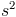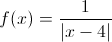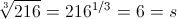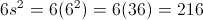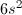Explanation:

First, you should put the equation in slope intercept form (y = mx + b), where m is the slope.

10x + 4y = 20

Isolate the y term

10x + 4y – 10x = 20 – 10x

4y = 20 – 10x

Rearrange the terms

4y = –10x + 20

Divide both sides by 4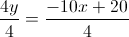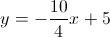The slope of the given line is –10/4. A perpendicular line will have a slope with the opposite reciprocal. In simpler terms, you flip the slope and change the sign, therefore, the opposite reciprocal is 4/10.

### Example Question #2 : How To Find The Slope Of Perpendicular Lines

Find the slope of the line that is perpendicular to the line that contains (1, 9) and (3, 4).

2/5

–5/2

–2/5

5/2

4/3

2/5

Explanation:

The slope of the line that contains the points (1, 9) and (3, 4) is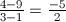.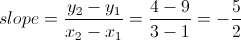The negative reciprocal is, which is the slope of the perpendicular line.

### Example Question #3 : How To Find The Slope Of Perpendicular Lines

If the slope of a line is equal to, what is the slope of its perpendicular intercept?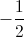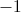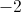Explanation:

Slope of lines that are perpendicular to each other are the negative reciprocal.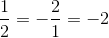### Example Question #4 : How To Find The Slope Of Perpendicular Lines

Two lines are perpendicular to each other. One of the lines is depicted by the equation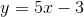. What is the slope of the other line?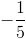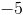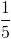Explanation:

Perpendicular lines have slopes that are negative reciprocals of one another. Since the original line has a slope of, the perpendicular line must have a slope of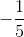.

### Example Question #5 : How To Find The Slope Of Perpendicular Lines

Two lines are perpendicular to each other. One of the lines' equations is.

What is the slope of the other line?Explanation:

Perpendicular lines have slopes that are negative reciprocals of one another. The given line's slope is 5, which means that the slope of the other line must be its negative reciprocal. The negative reciprocal of 5 is.

### Example Question #1 : How To Find The Slope Of Perpendicular Lines

What is the slope of a line that is perpendicular to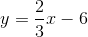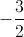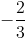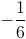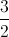Explanation:

Perpendicular lines have slopes that are negative inverses of one another. The slope of the given line is. The negative inverse ofis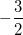, which must be the slope of the perpendicular line.

### Example Question #2 : How To Find The Slope Of Perpendicular Lines

What is the slope of a line that is perpendicular to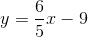?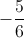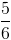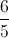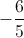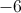Explanation:

The slopes of perpendicular lines are negative inverses of each other. The slope of the given line is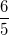. The negative inverse ofis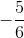.

### Example Question #8 : How To Find The Slope Of Perpendicular Lines

Find the slope of a line that's perpendicular to the following linear equation: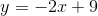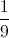none of theseExplanation:

We are givenTo find the slope that's perpendicular, we perform the following steps

1. First, take the slope from our original equation.  In our equation, the slope is2. Take the reciprocal of that slope.  The reciprocal is.
3. Finally, change the sign so that we end up with.  This is the number that represents the slope of the perpendicular line.

Another way to think of this problem is that the general formula for the slope that's perpendicular is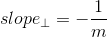whereis the slope of the original equation.  In our case,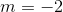. Thus,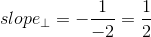### Example Question #3 : How To Find The Slope Of Perpendicular Lines

A line passes through points (–6,9) and (4,–4). Find the slope of the line that runs perpendicular to this line.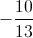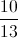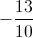Explanation:

To find the slope of this perpendicular line, we need to first find the slope of the line that passes through points (–6,9) and (4,–4). Remember, the general formula for slope is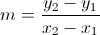, where the two points are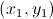and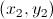.  In our case, we can calculate the slope using out two points.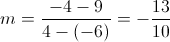The slope of the line passing through (–6,9) and (4,–4) is –13/10. To find the slope of the line that is perpendicular, just switch the sign and take the reciprocal of –13/10. This gives us 10/13. So 10/13 is the slope of that perpendicular line.

### Example Question #10 : How To Find The Slope Of Perpendicular Lines

Find the slope of the line perpendicular to the line that fits the following points:

(3,5), (2,7), (0,11)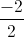Explanation:

1) To find the slope of the perpendicular line, we must first find the slope of the line fitting the given points. Slope is equal to change inover change in.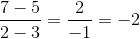2) The perpendicular slope is the opposite reciprocal of the slope of the line to which it is perpendicular. So flip the original slope upside down and multiply by.

Perpendicular slope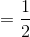← Previous 1 3 4 5